• 画饼图
2008-05-18 20:51:04

<%@ page language="java" contentType="image/png;charset=GB2312"
import="java.awt.*"
import="javax.imageio.*"
import="java.awt.geom.*"
import="java.awt.image.*"
import="java.text.*"
%>
<%!

// 绘制饼图的说明

public void drawTips(String tips, Color color, Arc2D.Double arc2d, Graphics2D g2d)
{
Arc2D.Double position = arc2d;

position.setAngleExtent(arc2d.getAngleExtent()/2);
position.x = arc2d.x - 15;
position.y = arc2d.y - 15;
position.width = arc2d.getWidth() + 30 ;
position.height = arc2d.getHeight() + 30;
Point2D.Double startPoint =
(Point2D.Double)position.getStartPoint();
Point2D.Double endPoint = (Point2D.Double)position.getEndPoint();

g2d.setPaint(color);

int stringLength = g2d.getFontMetrics().stringWidth(tips);

if (endPoint.x <= arc2d.getCenterX())
g2d.drawString(tips, (float)endPoint.x - stringLength, (float)endPoint.y);
else
g2d.drawString(tips, (float)endPoint.x , (float)endPoint.y );
}
%>

<%
// 清空缓冲区

response.reset();

// 注意这里的MIME类型

response.setContentType("image/png");

// 创建一个 500X375 的图像

int width = 500, height = 375;

BufferedImage image = new BufferedImage(width, height,
BufferedImage.TYPE_INT_RGB);

// 创建Java2D对象

Graphics2D g2d = image.createGraphics();

// 填充整个背景

g2d.setPaint(Color.WHITE);
g2d.fillRect(0, 0, width, height);

// 绘制阴影，由灰色渐进圆角矩形组成

width, height, new Color(218, 214, 212), false);
g2d.setPaint(grayGP);
RoundRectangle2D.Float bgRR =
new RoundRectangle2D.Float(5, 5, width-5, height-5 , 50, 50);
g2d.fill(bgRR);

// 绘制渐进蓝色圆角矩形背景

width/2, height/2, new Color(12, 104, 151), true);
g2d.setPaint(blueGP);
g2d.fillRoundRect(0, 0, width - 5, height - 5, 50, 50);

// 绘制深蓝色圆角矩形轮廓

BasicStroke bs = new BasicStroke(1.2f);
g2d.setStroke(bs);
g2d.setPaint(new Color(55,71, 105));
g2d.drawRoundRect(0, 0, width -5, height -5, 50, 50);

// 绘制图表标题

String chartTitle = "编程类图书销售量统计饼图";
g2d.setColor(Color.ORANGE);
g2d.setFont(new Font("汉鼎繁淡古", Font.PLAIN, 30));
int stringLength = g2d.getFontMetrics().stringWidth(chartTitle);
g2d.drawString(chartTitle, (width - stringLength) / 2, 25 );

// 定义圆弧

Arc2D.Double arc2d = new Arc2D.Double();

double startAngle = 30.0, arcAngle = 0.0;
double rectWidth = 320.0, rectHeight = 280.0;
Point2D.Double p2d = new Point2D.Double((width - rectWidth)/2, 70.0);
int arcType = Arc2D.PIE;

// 声明绘制数据

String bookTitle[] = {"Python", "JAVA", "C#", "Perl", "PHP"};
Color color[] = new Color;
color = new Color(99,99,0);
color = new Color(255,169,66);
color = new Color(33,255, 66);
color = new Color(33,0,255);
color = new Color(255,0,66);

double bookSales[] = new double;
double totalSales = 0.0 ;
double proportion = 0.0;

for (int i=0; i< bookSales.length; i++)
{
bookSales[i] = 1 + Math.random() * 99;
totalSales += bookSales[i];
}

for(int i = 0 ; i < bookTitle.length; i++)
{
arcAngle = bookSales[i]*360 / totalSales;
proportion = bookSales[i]/totalSales * 100 ;

g2d.setColor( color[i] );
arc2d = new Arc2D.Double(p2d.x, p2d.y, rectWidth,
rectHeight, startAngle, arcAngle, arcType);

// 填充圆弧

g2d.fill( arc2d) ;

// 描绘圆弧轮廓

g2d.setStroke(new BasicStroke(1.2f));
g2d.setPaint(Color.GRAY);
g2d.draw(arc2d);

// 更新圆弧的起始角度

startAngle += arcAngle;

// 格式化浮点数的输出格式

DecimalFormat twoDigits = new DecimalFormat("0.00");
g2d.setFont(new Font("Courier New", Font.PLAIN, 12));

// 绘制说明文字

drawTips(bookTitle[i] + " "+twoDigits.format(proportion) + "%",
Color.WHITE, arc2d, g2d);
}

// 部署图形

g2d.dispose();

// 利用ImageIO类的write方法对图像进行编码

ServletOutputStream sos = response.getOutputStream();
ImageIO.write(image, "PNG", sos);
sos.close();
%>PHP Perl
更多相关内容
• 2021年全国高校Python课程高级研修班（线上）中国大学MOOC“Python程序设计基础”第4次开课推荐图书：《Python程序设计（第3版）》，（ISBN：978-7-302-55...

中国大学MOOC“Python程序设计基础”第4次开课

推荐图书：

《Python程序设计（第3版）》，（ISBN：978-7-302-55083-9），董付国，清华大学出版社，2020年6月第1次印刷，2021年7月第9次印刷，山东省一流本科课程“Python应用开发”配套教材，清华大学出版社2020年度畅销图书（本书第二版为2019、2020年度畅销图书）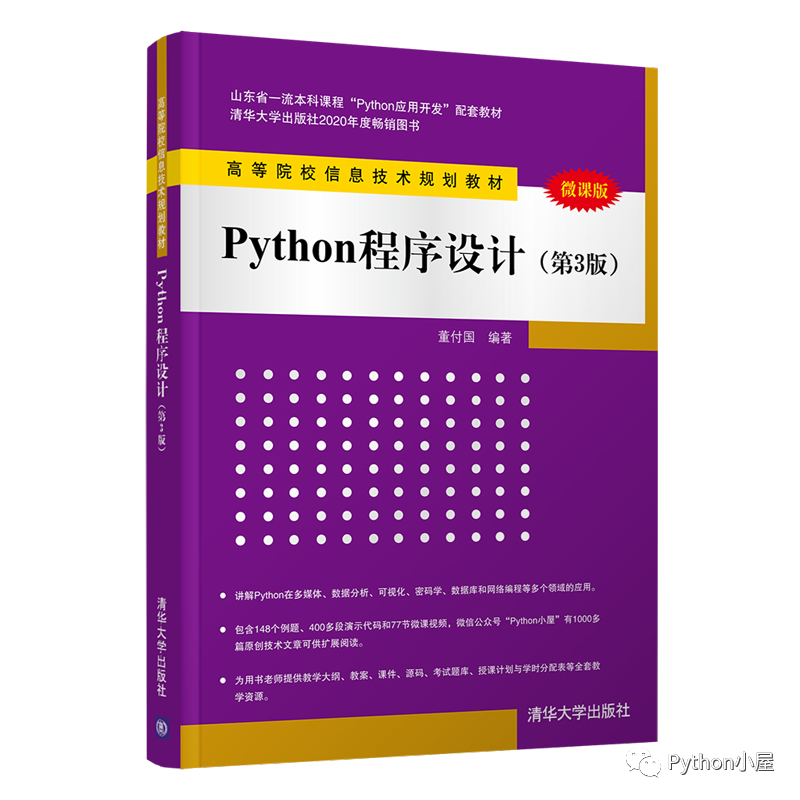京东购买链接：https://item.jd.com/12668739.html天猫、当当均有销售，可以选择自己常用平台搜索“董付国 第3版”找到本书。

配套资源：教学大纲、电子教案、微课视频、PPT课件、例题源码、习题答案、考试系统。

适用专业：计算机、网络工程、软件工程以及相关专业。

适用学时：>=64

页数：381

定价：59.8

微课视频：77个

目录（二级）

第1章  基础知识/1

1.1  如何选择Python版本

1.2  Python安装与简单使用

1.3  使用pip管理扩展库

1.4  Python基础知识

1.5  Python代码编写规范

1.6  Python文件名

1.7  Python程序的__name__属性

1.8  编写自己的包

1.9  Python快速入门

1.10  The Zen of Python

第2章  Python数据结构/31

2.1  列表

2.2  元组

2.3  字典

2.4  集合

2.5  其他数据结构

第3章  选择与循环/67

3.1  运算符与条件表达式

3.2  选择结构

3.3  循环结构

3.4  break和continue语句

3.5  综合运用

第4章  字符串与正则表达式/83

4.1  字符串

4.2  正则表达式

第5章  函数设计与使用/115

5.1  函数定义

5.2  形参与实参

5.3  参数类型

5.4  return语句

5.5  变量作用域

5.6  lambda表达式

5.7  案例精选

5.8  高级话题

第6章  面向对象程序设计/138

6.1  类的定义与使用

6.2  类的方法

6.3  属性

6.4  特殊方法与运算符重载

6.5  继承机制

第7章  文件操作/158

7.1  文件基本操作

7.2  文本文件基本操作

7.3  二进制文件操作

7.4  文件级操作

7.5  目录操作

7.6  案例精选

第8章  异常处理结构与程序调试/181

8.1  基本概念

8.2  Python异常类与自定义异常

8.3  Python中的异常处理结构

8.4  断言与上下文管理

8.5  使用IDLE调试代码

8.6  使用pdb模块调试程序

第9章  GUI编程/199

9.1  tkinter基础

9.2  tkinter精彩编程

第10章  网络程序设计/230

10.1  计算机网络基础知识

10.2  UDP和TCP编程

10.3  Socket编程案例精选

10.4  网页内容读取与网页爬虫

第11章  安卓平台的Python编程/248

11.1  QPython简介

11.2  安卓应用开发案例

第12章  Windows系统编程/254

12.1  注册表编程

12.2  创建可执行文件

12.3  调用外部程序

12.4  创建窗口

12.5  判断操作系统版本

12.6  系统运维

第13章  多线程与多进程编程/273

13.3  线程同步技术

13.4  多进程编程

第14章  数据库编程/294

14.1  SQLite应用

14.2  访问其他类型数据库

第15章  多媒体编程/306

15.1  图形编程

15.2  图像编程

15.3  音乐编程

15.4  语音识别

15.5  视频处理和摄像头接口调用

第16章  逆向工程与软件分析/328

16.1  主流项目与插件简介

16.2  IDAPython与Immunity Debugger编程

16.3  Windows平台软件调试原理

16.4  案例精选

第17章  科学计算与可视化/349

17.1  numpy简单应用

17.2  scipy简单应用

17.3  matplotlib简单应用

17.4  数据分析模块pandas

17.5  统计分析模块statistics

第18章  密码学编程/377

18.1  安全哈希算法

18.2  对称密钥密码算法DES和AES

18.3  非对称密钥密码算法RSA与数字签名算法DSA

=======================

任务描述：

使用pandas的DataFrame对象绘制饼状图，每列数据分别创建单独的轴域，然后使用matplotlib对已绘制的图形进行设置，设置饼状图中扇形外侧的文本标签，设置图例位置。

参考代码：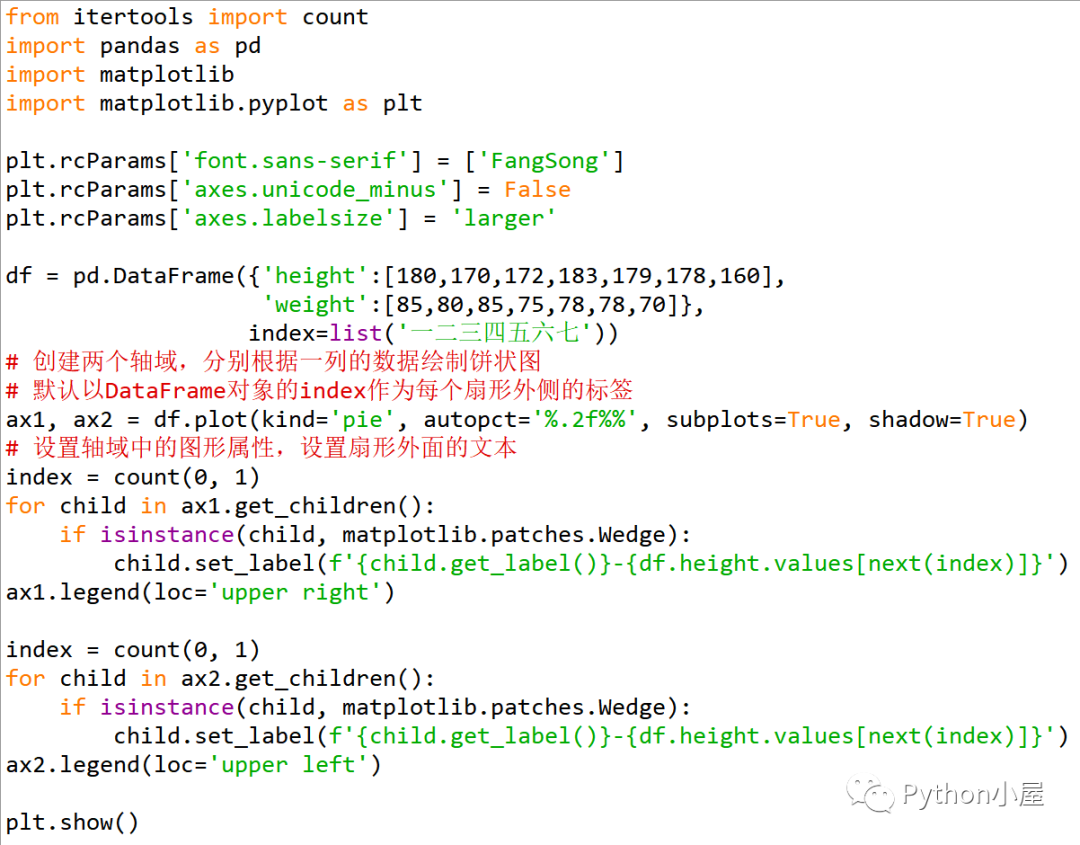运行结果：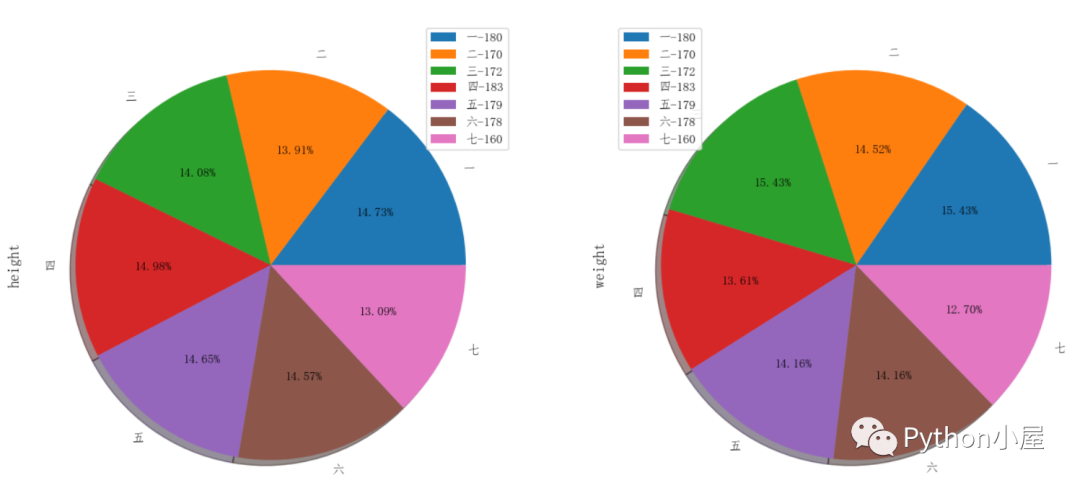温馨提示：

关注微信公众号“Python小屋”，在公众号后台发送消息“大事记”可以查看董付国老师与Python有关的重要事件；发送消息“教材”可以查看董付国老师出版的Python系列教材（已累计印刷超过120次）的适用专业详情；发送消息“历史文章”可以查看董付国老师推送的超过1000篇原创技术文章；发送消息“会议”或“培训”可以查看近期董付国老师的培训安排；发送消息“微课”可以查看董付国老师免费分享的超过500节Python微课视频；发送消息“课件”可以查看董付国老师免费分享的Python教学资源；发送消息“小屋刷题”可以下载“Python小屋刷题神器”，免费练习1401道客观题和329道编程题，题库持续更新；发送消息“编程比赛”了解Python小屋编程大赛详情。

展开全文编程语言 java epoll go
• 然后画饼图： y=price.values plt.figure(figsize=(5,5)) plt.title('计算机类畅销书籍价格占比',fontsize=15) patches,l_text,p_text=plt.pie(y,labels=data.index,autopct='%.1f %%',startangle=90,colors='...

爬虫新手小白的第一次“半独立”爬虫，为什么是“半独立”呢？因为基本的代码块是从其他博客借鉴过来的，在此基础上加入了自己的思考和实现。

（后面的价格获取感觉自己走了很多弯路，想到一步写一步，肯定有其他更直接的方法）

首先是导入需要的库（numpy和matplotlib是打算后续可视化用的）

#-*- coding:utf-8 -*-
import requests
from bs4 import BeautifulSoup as BS
#import numpy as np
#import matplotlib.pyplot as plt
import pandas as pd
import re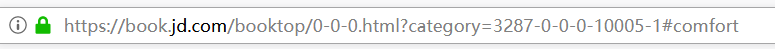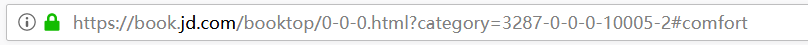畅销榜书籍一共有5页，每一页链接的不同在于最后的编号，可以用format函数

#初始索引页面链接
index = r"https://book.jd.com/booktop/0-0-0.html?category=3287-0-0-0-10001-{0}#comfort"
#获取页面内容
try:
r = requests.get(index)
r.raise_for_status()
r.encoding = r.apparent_encoding
return r.text
except:
print('请求失败')
#解析网页
def makeSoup(response):
soup = BS(response,'html.parser')
return soup
#获取每一页的网页链接
def createPagesList(soup):
urlList = []
baseUrl = r"https://book.jd.com/booktop/0-0-0.html?category=3287-0-0-0-10001-{0}#comfort"
pageindex = soup.select('.p-wrap .p-num a')
#获取分页中最后一页的页码
num = int(pageindex[-2].text)
for i in range(1,num+1,1):
#生成网页链接
url = baseUrl.format(str(i))
urlList.append(url)
return urlList
#还是获取每一页的网页链接，参数为初始索引链接，避免运行顺序出错
def getUrls(index):
soup = makeSoup(res)
urllist = createPagesList(soup)
return urllist

查看页面源代码知书籍信息所在类和标签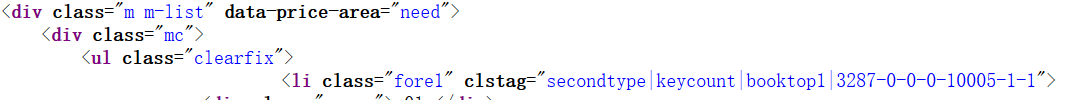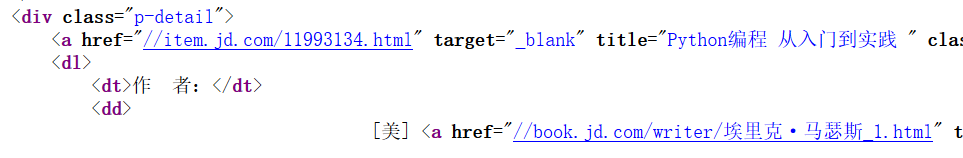#获取相关的书籍信息
def parseBooks(soup):
books = []
bookInfo = soup.select('.mc .clearfix li')
for book in bookInfo:
bookName = book.select('.p-detail a')['title']
bookAuthor = book.select('.p-detail dl dd a')['title']
#有些书名后面带有（与书名无关的内容）
bookName = bookName.split('（')
bookName = bookName.split('(')
bookName = bookName.split('【')

#将书名和出版社保存到列表中
books.append(bookName)
books.append(bookAuthor)

return books
#在循环中分别获取每个页面的书本信息，合并到一个列表中
pagelist = getUrls(index)
booklist = []
for url in pagelist:
soup = makeSoup(res)
books = parseBooks(soup)
booklist.extend(books)
bookname= []
bookauthor = []

for i in range(0,len(booklist),2):
name = booklist[i]
author = booklist[i+1]
#price = booklist[i+2]
bookname.append(name)
bookauthor.append(author)

查看价格信息时发现价格为空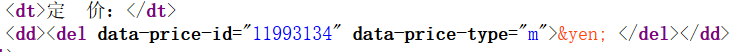查了很久资料，原来价格动态加载，要想服务器请求

我用的是火狐浏览器，右键点击查看元素，点击网络然后刷新页面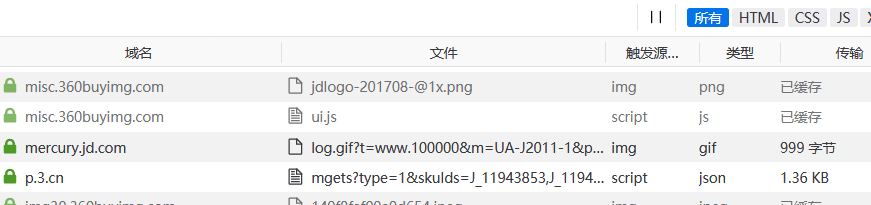在上面的文件中找到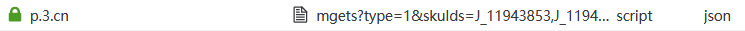点击消息头可以看到请求网址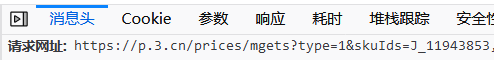点击响应可以看到书籍的信息，id是每本书的id号，p是价格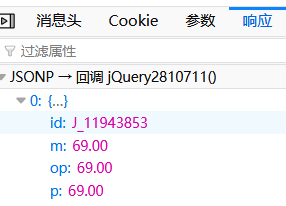观察发现，每本书的价格请求链接只有后面的‘skuIds’不同，而且就是书籍的id号，我的想法是先获得每一本书的id号，然后获得每一本书的价格请求链接，接着获取每一本书的价格。

想要获取每本书的id号，看看能不能从源代码中发现什么，果然在a标签的href属性中有一个链接，里面就包含了id号，把它提取出来，再利用正则表达式提取id就行啦！！！（说起来简单，可是花费我老多时间了.....哇）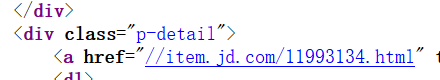下面来看获取id的代码

#获取每本一书的id号，存入一个列表
def get_bookid(soup):
idlists = []
bookids = soup.select('.mc .clearfix li')
for bookid in bookids:
bklist = bookid.select('.p-detail a')['href']
idlists.append(bklist)
lists = []
for idlist in idlists:
idl= re.findall(r"\d{8}",idlist)   #正则表达式匹配id
lists.append(idl)
return lists

得到的id是列表形式的，需要转化为字符串

BookId = []
pagelist = getUrls(index)
for url in pagelist:
soup = makeSoup(res)
lists0 = get_bookid(soup)
lists = sum(lists0,[])   #将列表里嵌套的列表元素转化为字符串形式
for i in range(0,20):
BookId.append(lists[i])

获得id之后，放入请求链接的尾部，还是用format()

price_reqs = []
price_urlList = []
base_priceUrl = 'https://p.3.cn/prices/mgets?&skuIds=J_{0}'  #请求价格链接，动态链接
for i in range(0,len(BookId),1):
price_url = base_priceUrl.format(BookId[i])
url_session = requests.Session()

price_req = url_session.get(price_url).text
price_reqs.append(price_req)

获得价格

bookPrice = []
for price_req in price_reqs:
bookPrice.append(re.findall(r'"p":"(.*?)"', price_req))

然后将书名作者价格读入pandas中，方便后续处理

#将数据读入pandas中
df_name = pd.DataFrame(bookname, columns=['书名'])
df_author = pd.DataFrame(bookauthor, columns=['作者'])
df_price = pd.DataFrame(bookPrice, columns=['京东价'])

df_book = df_name.join(df_author)
df_book = df_book.join(df_price)

到这里就差不多就完成啦，输出结果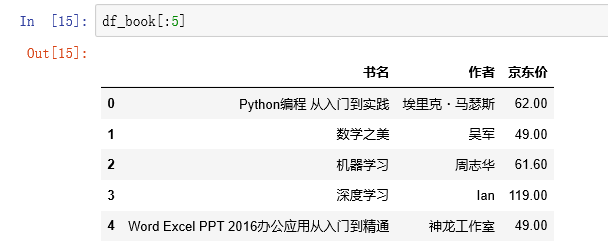最后还可以将数据保存到excel表格中

import xlrd
import xlwt

myxls=xlwt.Workbook(encoding='utf-8')
#sheet1.write(0,0,'书名')
#sheet1.write(0,1,'作者')
#sheet1.write(0,2,'京东价')
for i in range(0,len(bookname)):
sheet1.write(i,0,bookname[i])
sheet1.write(i,1,bookauthor[i])
sheet1.write(i,2,bookPrice[i])
myxls.save('books22.xlsx')由于爬取的书籍的数量和信息都很少，不好做可视化，简单地画一个饼图，意思一下

import numpy as np
import matplotlib.pyplot as plt

plt.rcParams['font.sans-serif']='SimHei' #设置中文字体为黑体，防止中文乱码
plt.rcParams['axes.unicode_minus']=False  #解决负号'-'显示为方块的问题

由于只有价格是数值型的，所以只对价格进行可视化。初始的价格类型为object类，直接画图会报错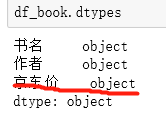修改价格的类型为浮点型：

df_book['京东价'] = df_book['京东价'].astype('float')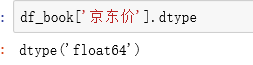首先用离散化函数cut()把价格分为几个区间：

price=pd.cut(df_book.京东价,[0.0,50.0,80.0,100.0,120.0,200.0]).value_counts()
price

然后画饼图：

y=price.values
plt.figure(figsize=(5,5))
plt.title('计算机类畅销书籍价格占比',fontsize=15)
patches,l_text,p_text=plt.pie(y,labels=data.index,autopct='%.1f %%',startangle=90,colors='rcmby')

for i in p_text:
i.set_size(10)
i.set_color('k')
for i in l_text:
i.set_size(10)
i.set_color('k')

plt.legend(loc='lower right', bbox_to_anchor=(1.5,0))

plt.show()

结果：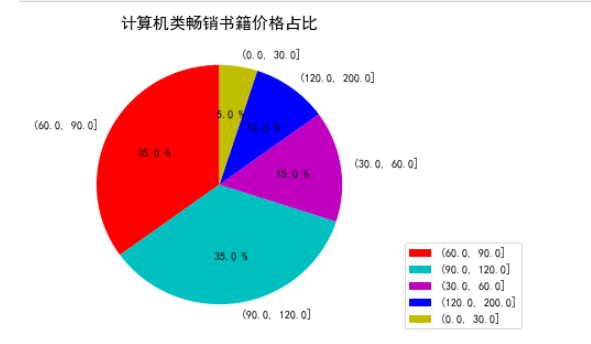待解决的问题：

每本书内容简介的爬取以及出版社的爬取，出版社的爬取与书名和作者类似，都是静态的，而内容简介与价格类似，都是动态。

1、爬取出版社遇到的问题：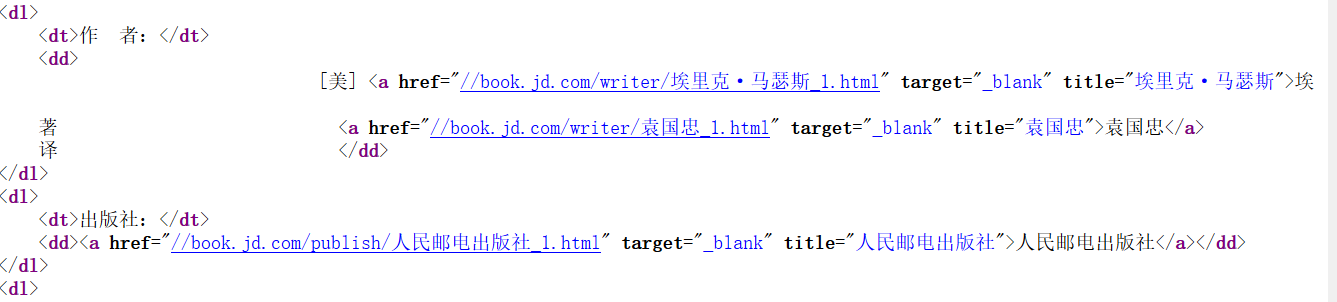作者和出版社的标签是相同的，用select方法提取，存储到一个列表中，

 bookAuthor = book.select('.p-detail dl dd a')['title']
bookPress = book.select('.p-detail dl dd a')['title']

问题是有的书籍中，作者有两个，一个著一个译，所以列表中索引就是第二个作者，那么出版社就是索引，可是有些书又只有一个作者，循环就用不了了，那应该怎么准确的写信息所在的标签呢？暂时没有找到解决的办法。只能得到如下结果：

出版社不能准确提取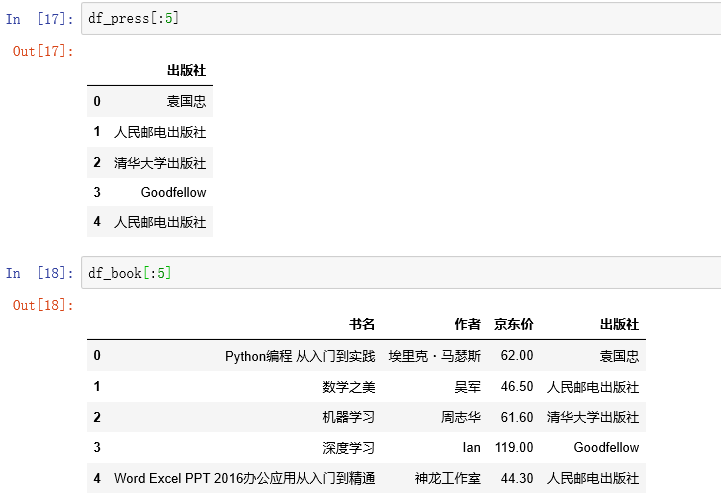2、爬取内容简介遇到的问题：

要获取内容简介部分的源代码，也是有一个动态的请求链接，方法与价格一样，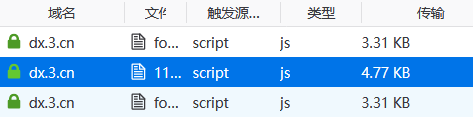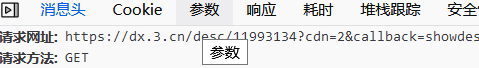select = etree.HTML(text)
#定位提取内容简介
content = select.xpath("//div/div/div//text()")

不会从以下源代码中提取内容简介（只能用xpath），用xpath能提取出来，又有个问题是不同书所在的xpath路径又不一样，不能所有书的内容简介一起提取，不知道该怎么办了。等再学习了网页的知识再看能不能解决。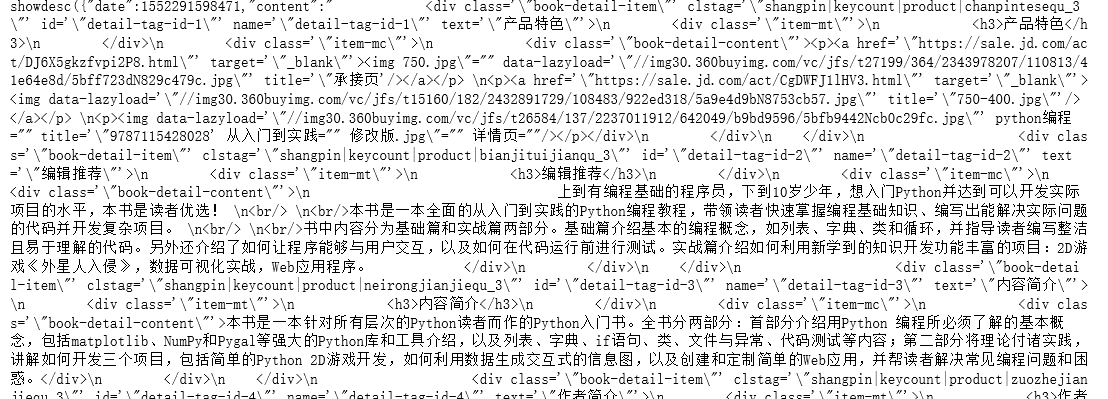这是爬一本书的结果。。。。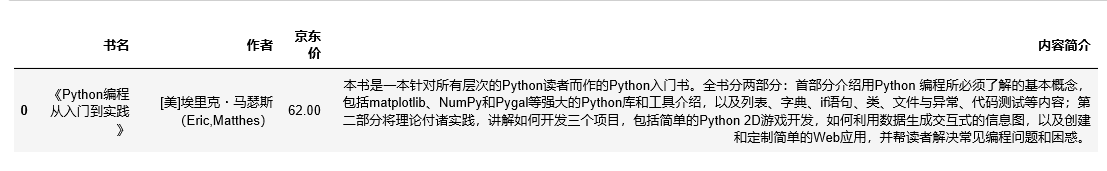展开全文• Python数据分析与挖掘实战》书籍笔记，包含每章节总结及对应代码，可供python数据分析与数据挖掘资料使用，另外包括数据挖掘比赛笔记总结。

## 一、书籍及源代码下载链接：

链接：https://pan.baidu.com/s/1oTde4_W1-0GcltkcGtG33A   提取码：1234

GitHub - GoAlers/python_data_analysis_and_mining_action: 《python数据分析与挖掘实战》的代码笔记

## 数据挖掘比赛笔记参考这篇：

数据挖掘比赛笔记总结_GoAl的博客-CSDN博客

### 一、Python数据分析工具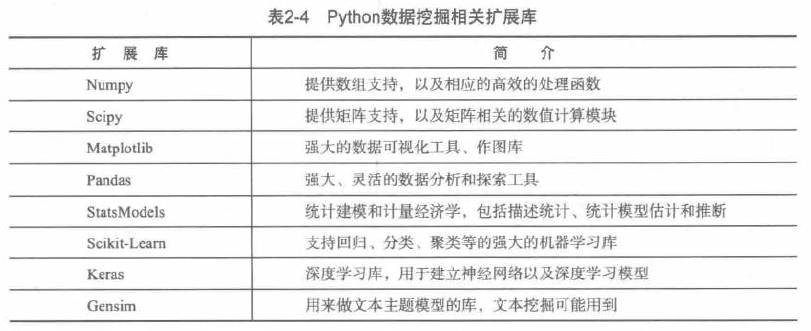### 二、数据探索

一、对数据的质量分析

异常值的分析：

1. 简单的统计量分析：查看最大最小值是否在合理范围

2.3δ原则，在正态分布下异常值被定义为一组定值与平均值的距离超过3倍的标准差。

3.箱形图分析：

异常值被定义为小于QL-1.5IQR 或大于QR+1.5IQR

QL是所有数据的下四分位，QR是所有数据的上四分位。IQR是QR-QL

DataFrame中describe()已经给出了基本的统计

二、数据特征分析

1.可以使用pandas、matplotlib绘制统计图

散点图矩阵可以分析每两个变量的关系。

2.计算相关系数

①Pearson相关系数

②Spearman秩相关系数

③判定系数

使用pandas的corr()计算相关系数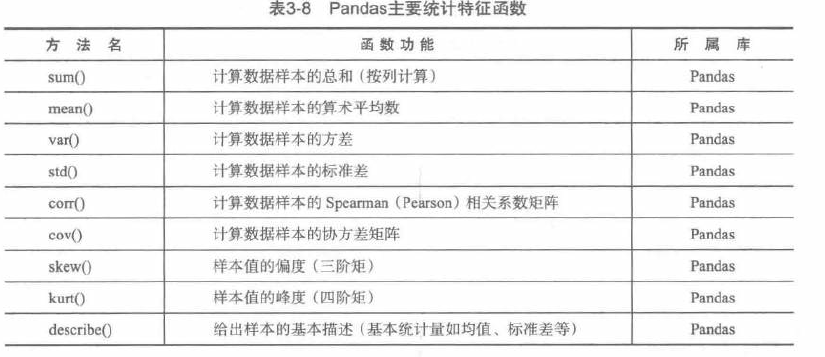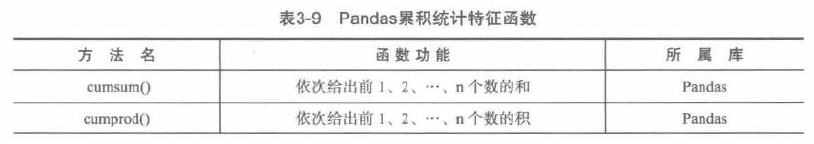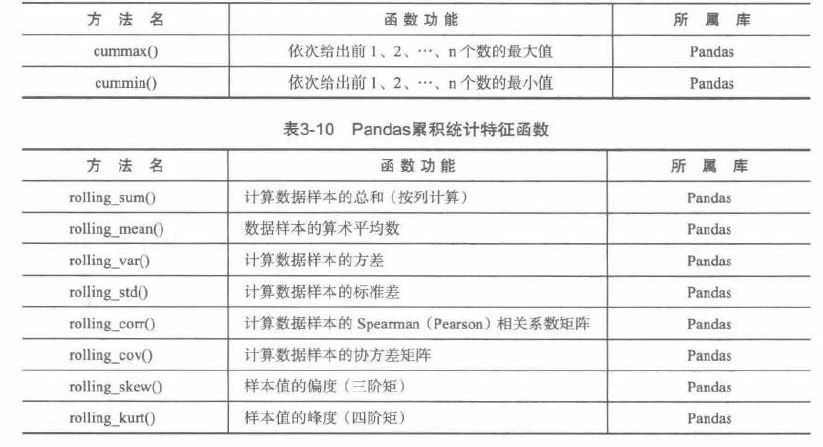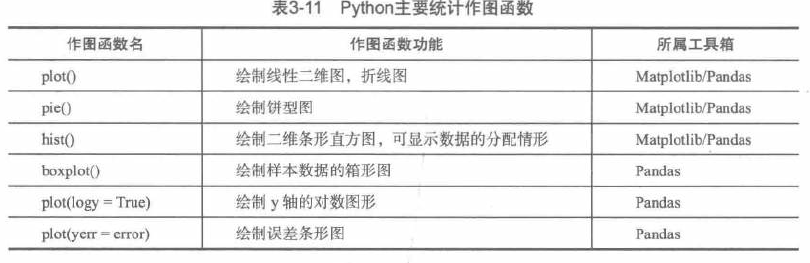绘制条形图和折线图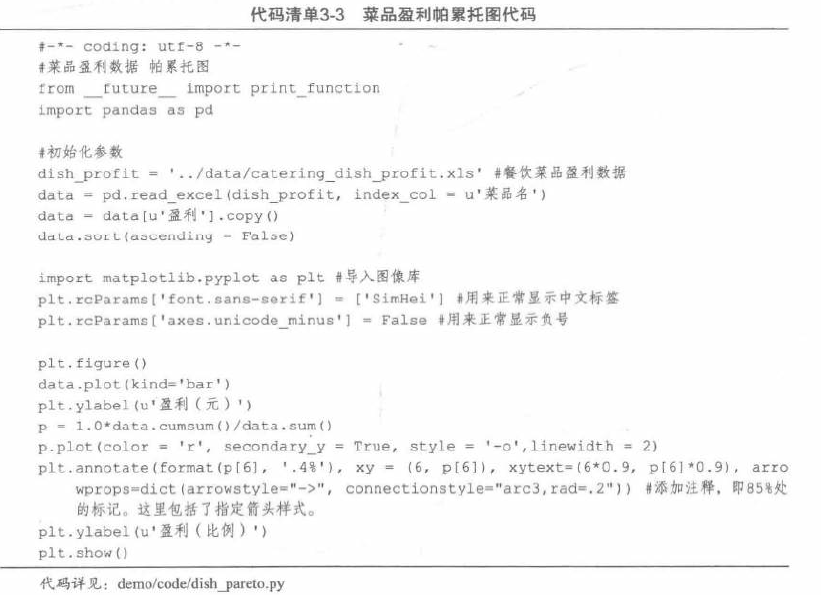### 三、数据预处理

缺失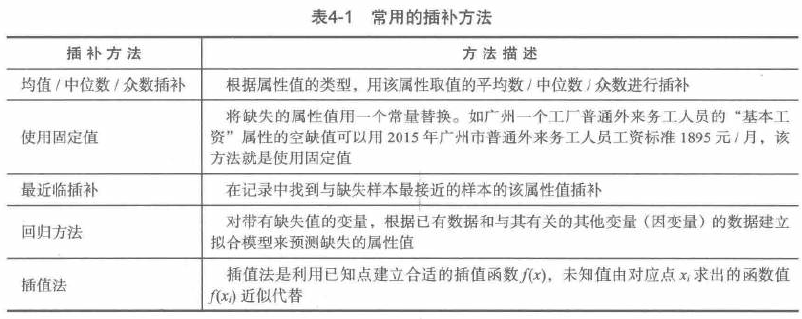## 二、Python数据分析入门

### Python入门

基本命令：

# for 循环
s = 0
for k in range(101):    #1-100
s = s + k
print s

# 函数
return x+2

return [x+2, y+2]   #返回列表

return x+3, y+3 #双重返回

# 匿名函数
f = lambda x : x+2  #定义函数f(x)= x+2
g = lambda x, y : x+y   #定义函数g(x,y)= x+y, g(1,2)结果为3

# 数据结构
# a, b是列表
# 列表函数cmp(a, b) len(a) max(a) min(a) sum(a) sorted(a)
# 列表对象方法 a.append(1) a.count(1) a.extend([1,2]) a.index(1) a.insert(2,1) a.pop(1)
b = a       # b是a的别名
b = a[:]    #数据复制

# 列表解析
a = [1, 2, 3]
b = []
for i in a:
b.append(i+2)
# 等价于
a =[1, 2, 3]
b =[i + 2 for i in a]

# 集合
d = {'today' : 20, "tomorrow" : 30} #创建
d['today']          #访问
# 其他创建方法
dict(['today', 20], ['tomorrow', 30])
dict.fromkeys(['today', 'tomorrow'], 20)

# 集合
s = {1, 2, 2, 4}
s = set([1,2,2,4])      #自动去除多余的值

# 函数式编程 lambda, map, reduce, filter
b = map(lambda x :x+2, a)
b = list(b);
#2.x中不需要，3.x中需要，因为map仅仅创建了一个待运行的命令容器，只有其他函数调用时才返回结果
# map命令将函数逐一运用到map列表的每个元素中，，最后返回一个数组，效率比for循环高一点

# reduce函数用于递归运算
reduce(lambda x, y: x*y, range(1, n+1))

# filter 用于筛选列表中符合条件的元素
b = filter(lambda x :x > 5 and x <8, range(10))
b = list(b)     # 同map

# 导入库
import math
math.sin(1)

import math as m
m.sin(1)

from math import exp as e
e(1)
sin(1)      #出错

from math import *      #直接导入，大量导入会引起命名冲突，不建议
exp(1)
sin(1)

# 导入future特征（2.x）
# 将print变为函数形式，即用print(a)格式输出
from __future__ import print_function
# 3.x中3/2=1.5, 3//2=1;2.x中3/2=1
from __future__ import division


### 第三方库

安装

Windows中

pip install numpy

或者下载源代码安装

python setup.py install

Pandas默认安装不能读写Excel文件，需要安装xlrd和xlwt库才能支持excel的读写

pip install xlrd
pip install xlwt

StatModel可pip可exe安装，注意，此库依赖于Pandas和patsy

Scikit-Learn是机器学习相关的库，但是不包含人工神经网络

 model.fit()     #训练模型，监督模型fit(X,y),非监督模型fit(X)

# 监督模型接口
model.predict(X_new)        #预测新样本
model.predict_proba(X_new)  #预测概率
model.score()               #得分越高，fit越好

# 非监督模型接口
model.transform()           #从数据中学到新的“基空间”
model.fit_transform()       #从数据中学到新的基，并按照这组基进行转换

Keras是基于Theano的强化的深度学习库，可用于搭建普通神经网络，各种深度学习模型，如自编码器，循环神经网络，递归神经网络，卷积神经网络。Theano也是一个Python库，能高效实现符号分解，速度快，稳定性好，实现了GPU加速，在密集型数据处理上是CPU的10倍，缺点是门槛太高。Keras的速度在Windows会大打折扣。

Windows下：安装MinGWindows--安装Theano---安装Keras--安装配置CUDA

Gensim用来处理语言方面的任务，如文本相似度计算、LDA、Word2Vec等，建议在Windows下运行。

Linux中

sudo apt-get install python-numpy
sudo apt-get install python-scipy
sudo apt-get install python-matplotlib

使用

Matplotlib默认字体是英文，如果要使用中文标签，

plt.rcParams['font.sans-serif'] = ['SimHei']

保存作图图像时，负号显示不正常：

plt.rcParams['axes.unicode_minus'] = False

## 三、数据探索

脏数据：缺失值、异常值、不一致的值、重复数据

### 异常值分析

1. 简单统计量分析：超出合理范围的值
2. 3sigma原则：若正态分布，异常值定义为偏差超出平均值的三倍标准差；否则，可用远离平均值的多少倍来描述。
3. 箱型图分析：异常值定义为小于Q_L-1.5IQR或者大于Q_U +1.5IQR。Q_L是下四分位数，全部数据有四分之一比他小。Q_U是上四分位数。IQR称为四分位数间距，IQR=Q_U-Q_L

  #-*- coding: utf-8 -*-
import pandas as pd

catering_sale = '../data/catering_sale.xls' #餐饮数据
data = pd.read_excel(catering_sale, index_col = u'日期') #读取数据，指定“日期”列为索引列

import matplotlib.pyplot as plt #导入图像库
plt.rcParams['font.sans-serif'] = ['SimHei'] #用来正常显示中文标签
plt.rcParams['axes.unicode_minus'] = False #用来正常显示负号

plt.figure() #建立图像
p = data.boxplot() #画箱线图，直接使用DataFrame的方法
x = p['fliers'].get_xdata() # 'flies'即为异常值的标签
y = p['fliers'].get_ydata()
y.sort() #从小到大排序，该方法直接改变原对象

#用annotate添加注释
#其中有些相近的点，注解会出现重叠，难以看清，需要一些技巧来控制。
#以下参数都是经过调试的，需要具体问题具体调试。
#xy表示要标注的位置坐标，xytext表示文本所在位置
for i in range(len(x)):
if i>0:
plt.annotate(y[i], xy = (x[i],y[i]), xytext=(x[i]+0.05 -0.8/(y[i]-y[i-1]),y[i]))
else:
plt.annotate(y[i], xy = (x[i],y[i]), xytext=(x[i]+0.08,y[i]))

plt.show() #展示箱线图

### 分布分析

定量数据的分布分析：求极差(max-min)，决定组距和组数，决定分点，列出频率分布表，绘制频率分布直方图。

定性数据的分布分析：饼图或条形图

### 统计量分析

集中趋势度量：均值、中位数、众数

离中趋势度量：极差、标准差、变异系数、四份位数间距

变异系数为：s表示标准差，x表示均值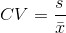#-*- coding: utf-8 -*-
#餐饮销量数据统计量分析
from __future__ import print_function
import pandas as pd

catering_sale = '../data/catering_sale.xls' #餐饮数据，一列为日期，一列为销量
data = pd.read_excel(catering_sale, index_col = u'日期') #读取数据，指定“日期”列为索引列
data = data[(data[u'销量'] > 400)&(data[u'销量'] < 5000)] #过滤异常数据
statistics = data.describe() #保存基本统计量
print(statistics)
print("--------------")

statistics.loc['range'] = statistics.loc['max']-statistics.loc['min'] #极差
statistics.loc['var'] = statistics.loc['std']/statistics.loc['mean'] #变异系数
statistics.loc['dis'] = statistics.loc['75%']-statistics.loc['25%'] #四分位数间距

print(statistics)

### 贡献度分析

又称帕累托分析，原理是帕累托法则，即20/80定律，同样的投入放在不同的地方会产生不同的收益。

  #-*- coding: utf-8 -*-
#菜品盈利数据 帕累托图
from __future__ import print_function
import pandas as pd

#初始化参数
dish_profit = '../data/catering_dish_profit.xls' #餐饮菜品盈利数据,菜品ID,菜品名 盈利
data = pd.read_excel(dish_profit, index_col = u'菜品名')
data = data[u'盈利'].copy()#保留两列数据
data.sort(ascending = False)

import matplotlib.pyplot as plt #导入图像库
plt.rcParams['font.sans-serif'] = ['SimHei'] #用来正常显示中文标签
plt.rcParams['axes.unicode_minus'] = False #用来正常显示负号

plt.figure()
data.plot(kind='bar')
plt.ylabel(u'盈利（元）')
p = 1.0*data.cumsum()/data.sum()
p.plot(color = 'r', secondary_y = True, style = '-o',linewidth = 2)
plt.annotate(format(p, '.4%'), xy = (6, p), xytext=(6*0.9, p*0.9),
#添加注释，即85%处的标记。这里包括了指定箭头样式。
plt.ylabel(u'盈利（比例）')
plt.show()

### 相关性分析

途径：绘制散点图、散点图矩阵、计算相关系数

Pearson相关系数：要求连续变量的取值服从正态分布。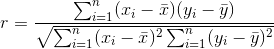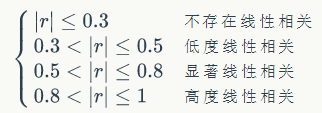相关系数r的取值范围[-1, 1]

Spearman相关系数：不服从正态分布的变量、分类或等级变量之间的关联性可用该系数，也称等级相关系数。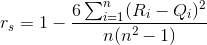对两个变量分别按照从小到大的顺序排序，得到的顺序就是秩。R_i表示x_i的秩次，Q_i表示y_i的秩次。

判定系数：相关系数的平方，用来解释回归方程对y的解释程度。

 #-*- coding: utf-8 -*-
#餐饮销量数据相关性分析
from __future__ import print_function
import pandas as pd

catering_sale = '../data/catering_sale_all.xls' #餐饮数据，含有其他属性
data = pd.read_excel(catering_sale, index_col = u'日期') #读取数据，指定“日期”列为索引列

data.corr() #相关系数矩阵，即给出了任意两款菜式之间的相关系数
data.corr()[u'百合酱蒸凤爪'] #只显示“百合酱蒸凤爪”与其他菜式的相关系数
data[u'百合酱蒸凤爪'].corr(data[u'翡翠蒸香茜饺']) #计算“百合酱蒸凤爪”与“翡翠蒸香茜饺”的相关系数

### 数据探索函数

方法名函数功能
D.sum()按列计算总和
D.mean()计算算数平均
D.var()方差
D.std()标准差
D.corr(method = ' pearson')Spearman(Pearson)相关系数矩阵
D.cov()协方差矩阵
D.skew()偏度(三阶矩)
D.kurt()峰度(四阶距)
D.describe()给出样本的基础描述

D = pd.DataFrame([range(1,8), range(2, 9)])
D.corr(method = 'spearman')     #计算相关系数矩阵
S1 = D.loc   #提取第一行
S2 = D.loc   #提取第二行
S1.corr(S2, method = 'pearson') #计算S1S2的相关系数

D = pd.DataFrame(np.random.randn(6, 5))     #产生6x5的表格
print D.cov()
print D.cov(D)  #计算第一列和第二列的方差
print D.skew()      #D是DataFrame或者Series
print D.describe()

方法名函数功能
cumsum()依次给出前1-n个数的和
cumprod()依次给出前1-n个数的积
cummax()依次给出前1-n个数的最大值
cummin()依次给出前1-n个数的最小值
方法名函数功能
rolling_sum()按列计算数据样本的总和
rolling_mean()算数平均数
rolling_var()方差
rolling_std()标准差
rolling_corr()相关系数矩阵
rolling_cov()协方差
rolling_skew()偏度
rolling_kurt()峰度
D = pd.Series(range(0,20))
print D.cumsum()

print pd.rolling_sum(D, 2)    #依次对相邻两项求和
方法名函数功能
plot()绘制线性二维图，折线图
pie()绘制饼形图
hist()绘制二维条形直方图，可现实数据的分配情形
boxplot()绘制箱型图
plot(logy = True)绘制y轴的对数图形
plot(yerr = error)绘制误差条形图


import matplotlib.pyplot as plt #导入图像库
plt.rcParams['font.sans-serif'] = ['SimHei'] #用来正常显示中文标签
plt.rcParams['axes.unicode_minus'] = False #用来正常显示负号
plt.figure(figsize = (7, 5))        #创建图像区域，指定比例
plt.show()                  #显示作图结果
############################################################################################
x = np.linspace(0, 2*np.pi, 50)
y = np.sin(x)
plt.plot(x, y, 'bp--')       #蓝色带星虚线plt.show()
############################################################################################
labels = 'Frogs', 'Hogs', 'Dogs', 'Logs'
sizes = [15, 30, 45, 10]     #每一块的比例
colors = ['yellowgreen', 'gold', 'lightskyblue', 'lightcoral']
explode = (0, 0.1, 0, 0)

plt.pie(sizes, explode = explode, labels = labels, colors = colors, autopct =
'%1.1f%%', shadow = True, startangle = 90)
plt.axis('equal')   #显示为圆
plt.show()
############################################################################################
x = np.random.randn(1000)       #1000个服从正态分布的随机数
plt.hist(x, 10)                 #分成10组
plt.show()
############################################################################################
plt.rcParams['font.sans-serif'] = ['SimHei'] #用来正常显示中文标签
plt.rcParams['axes.unicode_minus'] = False #用来正常显示负号

x = np.exp(np.arange(20))       #原始数据

plt.subplot(121)
plt.plot(range(0,20), x, label = u"原始数据图")
plt.legend()

plt.subplot(122)
plt.semilogy(range(0,20), x, label = u"对数数据图")
plt.legend()

plt.show()
############################################################################################
plt.rcParams['font.sans-serif'] = ['SimHei'] #用来正常显示中文标签
plt.rcParams['axes.unicode_minus'] = False #用来正常显示负号

error = np.random.random(10)        #定义误差条例
y = pd.Series(np.sin(np.arange(10)))
y.plot(yerr = error)
plt.show()


## 四、数据预处理

### 数据清洗

包括：删除原始数据中的无关数据、重复数据，平滑噪声数据，处理缺失值。
拉格朗日插值法：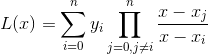当插值节点增减时，插值多项式就会发生变化，在实际计算中不方便。
牛顿插值法：P(x)是牛顿插值逼近函数，R(x)是误差函数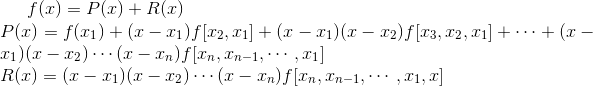Python的Scipy库中只提供了拉格朗日插值法的函数（实现上比较容易）

  #-*- coding: utf-8 -*-
# 插值时存在问题，不同的位置选取的数据点不一样，并且保证最后的数据是正确的
# 目前没有考虑连续脏数据的情况
#拉格朗日插值代码
import pandas as pd #导入数据分析库Pandas
from scipy.interpolate import lagrange #导入拉格朗日插值函数

inputfile = '../data/catering_sale.xls' #销量数据路径
outputfile = '../tmp/sales.xls' #输出数据路径

data[u'销量'][(data[u'销量'] < 400) | (data[u'销量'] > 5000)] = None #过滤异常值，将其变为空值

#自定义列向量插值函数
#s为列向量，n为被插值的位置，k为取前后的数据个数，默认为5,插值不要超过20
def ployinterp_column(s, n, k=5):
y = s[list(range(n-k, n)) + list(range(n+1, n+1+k))] #取数,y是长度为10的列表
y = y[y.notnull()] #剔除空值
return lagrange(y.index, list(y))(n) #插值并返回插值多项式，代入n得到插值结果

#逐个元素判断是否需要插值
k = 2
for i in data.columns:
for j in range(len(data)):
if (data[i].isnull())[j]: #如果为空即插值。
if (j >= k) and (j < len(data) - k):
y = data[i][list(range(j-k, j)) + list(range(j+1, j+1+k))] #取数,y是长度为10的列表
elif j < k :
y = data[i][list(range(0, j)) + list(range(j+1, 2 * k + 1))]
elif j >= len(data) - k:
y = data[i][list(range(len(data) - 1 - 2 * k, j)) + list(range(j+1, len(data)))]
y = y[y.notnull()] #剔除空值
data[i][j] = lagrange(y.index, list(y))(j) #插值并返回插值多项式，代入j得到插值结果

data.to_excel(outputfile) # 

### 数据集成

包括实体识别，冗余属性识别

### 数据变化

简单函数变换
规范化

1. 离差标准化(最小最大规范化)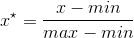1. 标准差标准化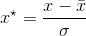1. 小数定标规范化：属性值映射在[-1, 1]之间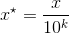#-*- coding: utf-8 -*-
#数据规范化
import pandas as pd
import numpy as np

datafile = '../data/normalization_data.xls' #参数初始化

print (data - data.min())/(data.max() - data.min()) #最小-最大规范化，按列出路
print (data - data.mean())/data.std() #零-均值规范化
print data/10**np.ceil(np.log10(data.abs().max())) #小数定标规范化

连续属性离散化

等宽法(至于相同宽度)、等频法(将相同数量的记录放进每个区间)、基于聚类分析的方法(K-means)

  import pandas as pd
from sklearn.cluster import KMeans #引入KMeans
import matplotlib.pyplot as plt
plt.rcParams['font.sans-serif'] = ['SimHei']     #用来正常显示中文标签
plt.rcParams['axes.unicode_minus'] = False      #用来正常显示负号

datafile = '../data/discretization_data.xls'    #参数初始化
data = data[u'肝气郁结证型系数'].copy()
k = 4       #分为4类

d1 = pd.cut(data, k, labels = range(k))     #等宽离散化，各个类比依次命名为0,1,2,3

#等频率离散化
w = [1.0*i/k for i in range(k+1)]           #为describe确定分位数0%,25%,50%,75%,100%
w = data.describe(percentiles = w)[4:4+k+1] #使用describe函数自动计算分位数并取出分位数
w = w*(1-1e-10)       #确保比最小值小
d2 = pd.cut(data, w, labels = range(k))

kmodel = KMeans(n_clusters = k, n_jobs = 1) #建立模型，n_jobs是并行数，一般等于CPU数较好
kmodel.fit(data.reshape((len(data), 1)))    #训练模型
c = pd.DataFrame(kmodel.cluster_centers_).sort(0) #输出聚类中心，并且排序（默认是随机序的）
w = pd.rolling_mean(c, 2).iloc[1:]          #相邻两项求中点，作为边界点
w =  + list(w) + [data.max()]         #把首末边界点加上
d3 = pd.cut(data, w, labels = range(k))

def cluster_plot(d, k):                     #自定义作图函数来显示聚类结果
plt.figure(figsize = (8, 3))
for j in range(0, k):
plt.plot(data[d==j], [j for i in d[d==j]], 'o')

plt.ylim(-0.5, k-0.5)
return plt

cluster_plot(d1, k).show()
cluster_plot(d2, k).show()
cluster_plot(d3, k).show()

属性构造：比如利用供入电量和供出电量计算线损率。

#-*- coding: utf-8 -*-
#线损率属性构造
import pandas as pd

#参数初始化
inputfile= '../data/electricity_data.xls' #供入供出电量数据
outputfile = '../tmp/electricity_data.xls' #属性构造后数据文件

data[u'线损率'] = (data[u'供入电量'] - data[u'供出电量'])/data[u'供入电量']

data.to_excel(outputfile, index = False) #保存结果

小波变换

用于非平稳信号的时频分析。基于小波变换的主要方法有：多尺度空间能量分布特征提取、多尺度空间的模极大值特征提取、小波包变换的特征提取、适应性小波神经网络的特征提取。
小波基函数：Harry小波基，db系列小波基，均值为0。积分为0.
小波变换：a是伸缩因子，b是平移因子，对小波基函数进行伸缩和平移变换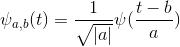任意函数f(t)的连续小波变换(CWT)为：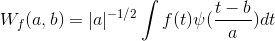在约束条件下有逆变换：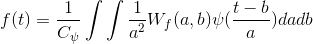python中scipy本身提供了信号处理函数，更好的信号处理库是PyWavelets(pywt)。

#小波特征变换提取代码
import pywt #导入PyWavelets

#参数初始化
inputfile= '../data/leleccum.mat'
#提取自Matlab的信号文件

signal = mat['leleccum']

coeffs = pywt.wavedec(signal, 'bior3.7', level = 5)
#返回结果为level+1个数字，第一个数组为逼近系数数组，后面的依次是细节系数数组

### 数据规约

属性规约：合并属性，逐步向前选择，逐步向后删除，决策树归纳，主成分分析。
主成分分析步骤：

1. 设原始变量X_1,X_2,..., X_p的n次观测数据矩阵为：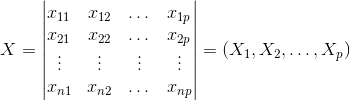1. 将数据矩阵按列进行中心标准化
2. 求相关系数矩阵R，$$R=(r_{ij})_{p\times p}$$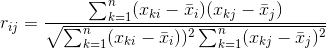其中，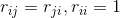1. 求R的特征方程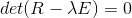的特征根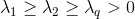1. 确定主成分个数m：alpha根据实际问题确定，一般取0.8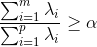1. 计算m个相应的单位特征向量：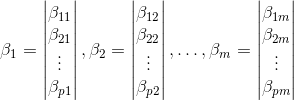1. 计算主成分：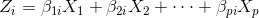import pandas as pd
from sklearn.decomposition import PCA

#参数初始化
inputfile = '../data/principal_component.xls'
outputfile = '../tmp/dimention_reducted.xls' #降维后的数据

pca = PCA()
pca.fit(data)
print pca.components_ #返回模型的各个特征向量
print pca.explained_variance_ratio_ #返回各个成分各自的方差百分比

#由上面可以看出前4个已经占了97%
pca = PCA(3)
pca.fit(data)
low_d = pca.transform(data)                 #降低唯独

pd.DataFrame(low_d).toexcel(outputfile)     #保存结果
pca.inverse_transform(low_d)        # 

数值规约：通过选择替代的、较小的数据来减少数据量。

### Python主要数据预处理函数

函数名函数功能
interpolate一维、高维数据插值
unique去除数据终端额重复数据
isnull判断是否空值
notnull判断是否非空值
PCA主成分分析
random生成随机矩阵

f = scipy.interpolate.lagrange(x,y) #一维数据的拉格朗日插值
f(2)            #计算插值结果
###################################################################
D = pd.Series([1,2,1,3,5])
D.unique()
np.uinque(D)        #这时候D可以是list,array,Series
###################################################################
D.isnull()      #D是series对象，返回布尔Series，D[D.isnull()]找到空值
###################################################################
np.random.randn(k,m,n)  #标准正态分布

## 五、挖掘建模

### 分类与预测

常用算法：回归分析、决策树、人工神经网络、贝叶斯网络、支持向量机。
Logistic回归
Logistic函数：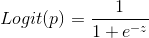回归模型：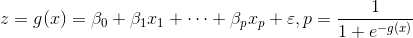#-*- coding: utf-8 -*-
#逻辑回归 自动建模
import pandas as pd
from sklearn.linear_model import LogisticRegression as LR
from sklearn.linear_model import RandomizedLogisticRegression as RLR

#参数初始化
filename = '../data/bankloan.xls'
x = data.iloc[:,:8].as_matrix()##变成矩阵
y = data.iloc[:,8].as_matrix()

rlr = RLR() #建立随机逻辑回归模型，筛选变量
rlr.fit(x, y) #训练模型
rlr.get_support() #获取特征筛选结果，也可以通过.scores_方法获取各个特征的分数
print(u'通过随机逻辑回归模型筛选特征结束')
#join() 表示连接，使用逗号，括号内必须是一个对象。如果有多个就编程元组，或是列表。
print(u'有效特征为：%s' % ','.join(data.columns[rlr.get_support()]))
x = data[data.columns[rlr.get_support()]].as_matrix() #筛选好特征

lr = LR() #建立逻辑货柜模型
lr.fit(x, y) #用筛选后的特征数据来训练模型
print(u'逻辑回归模型训练结束。')
print(u'模型的平均正确率为：%s' % lr.score(x, y)) #给出模型的平均正确率，本例为81.4%

Scikit-Learn提供了REF包可以用于特征消除。还提供了REFCV，可以通过交叉验证来对特征进行排序。
决策树
ID3、C4.5、CART算法
ID3：在决策树的各级节点上都用信息增益作为判断标准进行属性的选择，使得在每个节点上都能获得最大的类别分类增益，使分类后的额数据集的熵最小，这样使得树的平均深度最小，从而有效地提高了分类效率。
步骤：

1. 对当前样本集合，计算所有属性的信息增益
2. 选择信息增益最大的属性作为测试属性，把测试属性取值相同的样本划为同一个子样本集
3. 若子样本集的类别只有单个，则分支为叶节点；否则对子样本集循环调用本算法#-*- coding: utf-8 -*-
#使用ID3决策树算法预测销量高低
import pandas as pd
from sklearn.tree import export_graphviz
from sklearn.externals.six import StringIO
from sklearn.tree import DecisionTreeClassifier as DTC

#参数初始化
inputfile = '../data/sales_data.xls'
data = pd.read_excel(inputfile, index_col = u'序号') #导入数据

#数据是类别标签，要将它转换为数据
#用1来表示“好”、“是”、“高”这三个属性，用-1来表示“坏”、“否”、“低”
data[data == u'好'] = 1
data[data == u'是'] = 1
data[data == u'高'] = 1
data[data != 1] = -1
x = data.iloc[:,:3].as_matrix().astype(int)
y = data.iloc[:,3].as_matrix().astype(int)

dtc = DTC(criterion='entropy') #建立决策树模型，基于信息熵
dtc.fit(x, y) #训练模型

#导入相关函数，可视化决策树。
#导出的结果是一个dot文件，需要安装Graphviz才能将它转换为pdf或png等格式。
with open("tree.dot", 'w') as f:
f = export_graphviz(dtc, feature_names = ['tianqi', 'zhoumo', 'cuxiao'], out_file = f)
#f = export_graphviz(dtc, feature_names = [u'天气', u'周末', u'促销'], out_file = f)

#文本打开指定中文字体
#edge [fontname = "SimHei"];/*添加，指定中文为黑体*/
#node [fontname = "SimHei"];/*添加，指定中文为黑体*/
#安装Graphviz
#在命令行中编译

人工神经网络

  #-*- coding: utf-8 -*-
#使用神经网络算法预测销量高低

import pandas as pd

#参数初始化
inputfile = '../data/sales_data.xls'
data = pd.read_excel(inputfile, index_col = u'序号') #导入数据

#数据是类别标签，要将它转换为数据
#用1来表示“好”、“是”、“高”这三个属性，用0来表示“坏”、“否”、“低”
data[data == u'好'] = 1
data[data == u'是'] = 1
data[data == u'高'] = 1
data[data != 1] = 0
x = data.iloc[:,:3].as_matrix().astype(int)
y = data.iloc[:,3].as_matrix().astype(int)

from keras.models import Sequential
from keras.layers.core import Dense, Activation

model = Sequential() #建立模型

model.compile(loss = 'binary_crossentropy', optimizer = 'adam', class_mode = 'binary')
#编译模型。由于我们做的是二元分类，所以我们指定损失函数为binary_crossentropy，以及模式为binary
#另外常见的损失函数还有mean_squared_error、categorical_crossentropy等，请阅读帮助文件。

model.fit(x, y, nb_epoch = 1000, batch_size = 10) #训练模型，学习一千次
yp = model.predict_classes(x).reshape(len(y)) #分类预测

from cm_plot import * #导入自行编写的混淆矩阵可视化函数
cm_plot(y,yp).show() # 

算法评价：相对误差、均方误差、识别准确度、识别精确率、ROC曲线

### 聚类分析

K-Means算法

  #-*- coding: utf-8 -*-
#使用K-Means算法聚类消费行为特征数据

import pandas as pd
from sklearn.cluster import KMeans
import matplotlib.pyplot as plt
plt.rcParams['font.sans-serif'] = ['SimHei'] #用来正常显示中文标签
plt.rcParams['axes.unicode_minus'] = False #用来正常显示负号

#参数初始化
inputfile = '../data/consumption_data.xls' #销量及其他属性数据
outputfile = '../tmp/data_type.xls' #保存结果的文件名
k = 3 #聚类的类别
iteration = 500 #聚类最大循环次数
data = pd.read_excel(inputfile, index_col = 'Id') #读取数据
data_zs = 1.0*(data - data.mean())/data.std() #数据标准化

model = KMeans(n_clusters = k, n_jobs = 1, max_iter = iteration) #分为k类，并发数4
model.fit(data_zs) #开始聚类

#简单打印结果
r1 = pd.Series(model.labels_).value_counts() #统计各个类别的数目
r2 = pd.DataFrame(model.cluster_centers_) #找出聚类中心
r = pd.concat([r2, r1], axis = 1) #横向连接（0是纵向），得到聚类中心对应的类别下的数目
r.columns = list(data.columns) + [u'类别数目'] #重命名表头
print(r)        #打印分类中心和分类数量

#详细输出原始数据及其类别
r = pd.concat([data, pd.Series(model.labels_, index = data.index)], axis = 1)  #详细输出每个样本对应的类别
r.columns = list(data.columns) + [u'聚类类别'] #重命名表头
r.to_excel(outputfile) #保存分类结果

def density_plot(data): #自定义作图函数
p = data.plot(kind='kde', linewidth = 2, subplots = True, sharex = False)
[p[i].set_ylabel(u'密度') for i in range(k)]
plt.legend()
return plt

pic_output = '../tmp/pd_' #概率密度图文件名前缀
for i in range(k):
density_plot(data[r[u'聚类类别']==i]).savefig(u'%s%s.png' %(pic_output, i))

聚类算法评价：purity评价法、RI评价法、F值评价法

对象名函数功能
KMeansK均值聚类
AffinityPropagation吸引力传播聚类
SpectralClustering谱聚类，由于KMeans
AgglomerativeClustering层次聚类
DBSCAN具有噪声的基于密度的聚类算法
MeanShift均值漂移聚类算法
BIRCH层次聚类算法，可以处理大规模数据

先用对应的函数建立模型，然后使用fit方法训练模型，之后用label_方法给出样本数据的标签，或者用predict方法预测新的输入的标签。

TENSE：提供一种有效地数据降维的方式，在2维或者3维战士聚类结果。

  #-*- coding: utf-8 -*-
#接k_means.py
from sklearn.manifold import TSNE
import matplotlib.pyplot as plt
plt.rcParams['font.sans-serif'] = ['SimHei'] #用来正常显示中文标签
plt.rcParams['axes.unicode_minus'] = False #用来正常显示负号

tsne = TSNE()
tsne.fit_transform(data_zs) #进行数据降维
tsne = pd.DataFrame(tsne.embedding_, index = data_zs.index) #转换数据格式

#不同类别用不同颜色和样式绘图
d = tsne[r[u'聚类类别'] == 0]
plt.plot(d, d, 'r.')
d = tsne[r[u'聚类类别'] == 1]
plt.plot(d, d, 'go')
d = tsne[r[u'聚类类别'] == 2]
plt.plot(d, d, 'b*')
plt.show()

### 关联分析

常用算法：Apriori、FP-Tree、Eclt算法、灰色关联法

Ariori算法

支持度：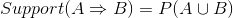,A、B同时发生的概率

置信度：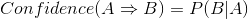A发生B发生的概率
同时满足最小支持度和最小置信度称满足强规则
算法步骤：

1. 扫描事物集，得到没个候选项的支持度
2. 比较候选支持度与最小支持度，得到1项频繁集L_1
3. 由L_1产生候选项集C_2，并计算支持度
4. 比较候选支持度和最小支持度，得到2项频繁集L_2
5. 类推，直至不能产生新的候选项集

  #-*- coding: utf-8 -*-
from __future__ import print_function
import pandas as pd

#自定义连接函数，用于实现L_{k-1}到C_k的连接
def connect_string(x, ms):
x = list(map(lambda i:sorted(i.split(ms)), x))
l = len(x)
r = []
for i in range(len(x)):
for j in range(i,len(x)):
if x[i][:l-1] == x[j][:l-1] and x[i][l-1] != x[j][l-1]:
r.append(x[i][:l-1]+sorted([x[j][l-1],x[i][l-1]]))
return r

#寻找关联规则的函数
def find_rule(d, support, confidence, ms = u'--'):
result = pd.DataFrame(index=['support', 'confidence']) #定义输出结果

support_series = 1.0*d.sum()/len(d) #支持度序列
column = list(support_series[support_series > support].index) #初步根据支持度筛选
k = 0

while len(column) > 1:
k = k+1
print(u'\n正在进行第%s次搜索...' %k)
column = connect_string(column, ms)
print(u'数目：%s...' %len(column))
sf = lambda i: d[i].prod(axis=1, numeric_only = True) #新一批支持度的计算函数

#创建连接数据，这一步耗时、耗内存最严重。当数据集较大时，可以考虑并行运算优化。
d_2 = pd.DataFrame(list(map(sf,column)), index = [ms.join(i) for i in column]).T

support_series_2 = 1.0*d_2[[ms.join(i) for i in column]].sum()/len(d) #计算连接后的支持度
column = list(support_series_2[support_series_2 > support].index) #新一轮支持度筛选
support_series = support_series.append(support_series_2)
column2 = []

for i in column: #遍历可能的推理，如{A,B,C}究竟是A+B-->C还是B+C-->A还是C+A-->B？
i = i.split(ms)
for j in range(len(i)):
column2.append(i[:j]+i[j+1:]+i[j:j+1])

cofidence_series = pd.Series(index=[ms.join(i) for i in column2]) #定义置信度序列

for i in column2: #计算置信度序列
cofidence_series[ms.join(i)] = support_series[ms.join(sorted(i))]/support_series[ms.join(i[:len(i)-1])]

for i in cofidence_series[cofidence_series > confidence].index: #置信度筛选
result[i] = 0.0
result[i]['confidence'] = cofidence_series[i]
result[i]['support'] = support_series[ms.join(sorted(i.split(ms)))]

result = result.T.sort(['confidence','support'], ascending = False) #结果整理，输出
print(u'\n结果为：')
print(result)

return result
#######################################################33
#-*- coding: utf-8 -*-
#使用Apriori算法挖掘菜品订单关联规则
from __future__ import print_function
import pandas as pd
from apriori import * #导入自行编写的apriori函数

outputfile = '../tmp/apriori_rules.xls' #结果文件

print(u'\n转换原始数据至0-1矩阵...')
ct = lambda x : pd.Series(1, index = x[pd.notnull(x)]) #1表示逐行转换。转换0-1矩阵的过渡函数
b = map(ct, data.as_matrix()) #用map方式执行,b是list
data = pd.DataFrame(b).fillna(0) #空值用0填充
print(u'\n转换完毕。')
del b #删除中间变量b，节省内存

support = 0.2 #最小支持度
confidence = 0.5 #最小置信度
ms = '---' #连接符，默认'--'，用来区分不同元素，如A--B。需要保证原始表格中不含有该字符

find_rule(data, support, confidence, ms).to_excel(outputfile) #保存结果

### 时序模式

非平稳时间序列分析：许多非平稳序列差分后会显示出平稳序列的性质，这时称之为差分平稳序列，可以先做差分然后用ARMA模型进行拟合。这种方法称之为ARIMA模型。

  #-*- coding: utf-8 -*-
#arima时序模型

import pandas as pd
import matplotlib.pyplot as plt
from statsmodels.graphics.tsaplots import plot_acf
from statsmodels.graphics.tsaplots import plot_pacf
from statsmodels.stats.diagnostic import acorr_ljungbox
from statsmodels.tsa.arima_model import ARIMA
plt.rcParams['font.sans-serif'] = ['SimHei'] #用来正常显示中文标签
plt.rcParams['axes.unicode_minus'] = False #用来正常显示负号

#参数初始化
discfile = '../data/arima_data.xls'
forecastnum = 5

#读取数据，指定日期列为指标，Pandas自动将“日期”列识别为Datetime格式
data = pd.read_excel(discfile, index_col = u'日期')

#时序图
data.plot()
plt.show()
plt.title('Time Series')

#自相关图
plot_acf(data).show()

#平稳性检测

#差分后的结果
D_data = data.diff().dropna()
D_data.columns = [u'销量差分']
D_data.plot() #时序图
plt.show()
plot_acf(D_data).show() #自相关图
plot_pacf(D_data).show() #偏自相关图

#白噪声检验
print(u'差分序列的白噪声检验结果为：', acorr_ljungbox(D_data, lags=1)) #返回统计量和p值

data[u'销量'] = data[u'销量'].astype(float)
#定阶
pmax = int(len(D_data)/10) #一般阶数不超过length/10
qmax = int(len(D_data)/10) #一般阶数不超过length/10
bic_matrix = [] #bic矩阵
for p in range(pmax+1):
tmp = []
for q in range(qmax+1):
try: #存在部分报错，所以用try来跳过报错。
tmp.append(ARIMA(data, (p,1,q)).fit().bic)
except:
tmp.append(None)
bic_matrix.append(tmp)

bic_matrix = pd.DataFrame(bic_matrix) #从中可以找出最小值

p,q = bic_matrix.stack().idxmin() #先用stack展平，然后用idxmin找出最小值位置。
print(u'BIC最小的p值和q值为：%s、%s' %(p,q))
model = ARIMA(data, (p,1,q)).fit() #建立ARIMA(0, 1, 1)模型
model.summary2() #给出一份模型报告
model.forecast(5) #作为期5天的预测，返回预测结果、标准误差、置信区间。

函数名函数功能
acf计算自相关系数
plot_acf画自相关系数图
pacf计算偏相关系数
plot_pacf画图
diff差分运算
ARIMA创建模型
summary给出ARIMA模型的报告
aic/bic/hqic计算ARIMA模型的指标
forecast预测
acorr_ljungboxLjung-Box检验，是否白噪声

  autocorr = acf(data, unbiased = False, nlags = 40, qstat = False, fft = False, alpha = False)
# data 为观测值序列(时间序列)，可以是DataFrame或者Series

h = adfuller(Series, maxlag = None, Regression = 'c', autolog = 'AIC', store = False, regresults =False)

D.diff()        #D为Pandas的DataFrame或Series

arima = ARIMA(data, (p, 1, q)).fit()        #data为输入的时间序列，p,q为对应的阶

amima.summary()         #返回一份格式化的模型报告

arima.bic

a,b,c = arima.forecast(num)     #num为要预测的天数,a为返回的预测值,b为预测误差,c为置信区间

### 离群点检测

方法：基于统计、基于邻近度、基于密度、基于聚类。
基于统计：一元正态分布若数据点在3倍标准差之外。
混合模型的离群点检测：数据的统计分布未知或者没有训练数据可用，很难建立模型。
基于原型的聚类：聚类所有的对象，然后评估对象属于簇的程度。如果删除一个对象导师制该目标显著改进，则可将该对象视为离群点。离群点可能形成小簇从而逃避检测。

  #-*- coding: utf-8 -*-
#使用K-Means算法聚类消费行为特征数据
import numpy as np
import pandas as pd
from sklearn.cluster import KMeans
import matplotlib.pyplot as plt

plt.rcParams['font.sans-serif'] = ['SimHei'] #用来正常显示中文标签
plt.rcParams['axes.unicode_minus'] = False #用来正常显示负号

#参数初始化
inputfile = '../data/consumption_data.xls' #ID 和三个属性
k = 3 #聚类的类别
threshold = 2 #离散点阈值
iteration = 500 #聚类最大循环次数
data = pd.read_excel(inputfile, index_col = 'Id') #读取数据
data_zs = 1.0*(data - data.mean())/data.std() #数据标准化

model = KMeans(n_clusters = k, n_jobs = 1, max_iter = iteration) #分为k类，并发数4
model.fit(data_zs) #开始聚类

#标准化数据及其类别
r = pd.concat([data_zs, pd.Series(model.labels_, index = data.index)], axis = 1)  #每个样本对应的类别
r.columns = list(data.columns) + [u'聚类类别'] #重命名表头

norm = []
for i in range(k): #逐一处理
norm_tmp = r[['R', 'F', 'M']][r[u'聚类类别'] == i]-model.cluster_centers_[i]
norm_tmp = norm_tmp.apply(np.linalg.norm, axis = 1) #求出绝对距离
norm.append(norm_tmp/norm_tmp.median()) #求相对距离并添加

norm = pd.concat(norm) #合并

norm[norm <= threshold].plot(style = 'go') #正常点

discrete_points = norm[norm > threshold] #离群点
discrete_points.plot(style = 'ro')

for i in range(len(discrete_points)): #离群点做标记
id = discrete_points.index[i]
n = discrete_points.iloc[i]
plt.annotate('(%s, %0.2f)'%(id, n), xy = (id, n), xytext = (id, n))#有标注的点是离群点

plt.xlabel(u'编号')
plt.ylabel(u'相对距离')
plt.show()

## 电力窃漏电用户自动识别

数据分析：

1. 分布分析：用户类别窃漏电情况分布发现，非居民类不存在漏电情况。故可清理
2. 周期性分析：找到一个正常的用户和漏电用户，分别观察规律。发现正常用户有明显的周期性。

数据预处理

1. 数据清洗：过滤掉非居民类的数据和节假日数据。
2. 缺失值处理：拉格朗日插补法进行插补
3. 数据变换：用电趋势指标、5天平均线损率、告警指标计数

模型构建

1. 用LM神经网络和CART决策树模型建模
2. ROC曲线比较性能

## 航空公司客户价值分析

数据分析：缺失值分析和异常值分析，异常值看最大和最小值

数据预处理：

1. 数据清洗，丢弃缺失值、票价为0折扣率不为0的数据
2. 属性规约，删除不相关或者弱相关属性
3. 数据变换：计算指标，并对数据进行标准化处理

模型构建

1. K-Means算法对客户数据进行分群，分为5类。
2. 结合图表对结果进行分析

## 中医证型关联规则挖掘

数据预处理

1. 数据清洗：删除整理无效问卷
2. 属性规约：将冗余属性和无关属性删除
3. 数据变换：构造属性，并将属性离散化

模型构建

采用Apriori关联规则算法对模型的样本数据进行分析，以模型参数设置的最小支持度和最小置信度作为条件，输出关联规则结果。

## 基于水色图像的水质评价

数据预处理

1. 图像切割：提取水样图像中间部分具有代表意义的图像
2. 特征提取：颜色的一阶、二阶、三阶矩

模型构建

为提高区分度，将所有特征乘以常数k。然后建立支持向量机模型。

水质评价

对新增的水质图像作评价。

## 家用电器用户行为分析与事件识别

数据预处理

1. 数据规约：去除无用的属性和状态
2. 数据变换：确定用水事件的阈值
3. 数据清洗

模型构建：训练神经网络

模型检验：使用测试数据

## 应用系统负载分析与磁盘容量预测

数据分析：通过时序图观察数据的平稳性和周期性

数据预处理

1. 数据清洗：删除重复值
2. 属性构造：合并属性

模型构建

1. 检验平稳性，单位根检验
2. 白噪声检验
3. 模型识别：采用极大似然比方法进行模型的参数估计，采用BIC信息准则对模型进行定阶。ARIMA(0,1,1)
4. 模型检验：检验模型残差序列是否为白噪声如果不是，说明还有未提取的有用信息，需要修改模型。

模型评价：计算平均绝对误差，均方根误差

## 电子商务网站用户行为分析及服务推荐

数据抽取：建立数据库--导入数据--搭建Python数据库操作环境

数据分析

1. 网页类型分析
2. 点击次数分析
3. 网页排名

数据预处理

1. 数据清洗：删除数据(中间页面网址、发布成功网址、登录助手页面)
2. 数据变化：识别翻页网址并去重，错误分类网址手动分类，并进一步分类
3. 属性规约：只选择用户和用户选择的网页数据

模型构建

基于物品的协同滤波算法：计算物品之间的相似度，建立相似度矩阵；根据物品的相似度和用户的历史行为给用户生成推荐列表。

相似度计算方法：夹角余弦、Jaccard系数、相关系数

## 财政收入影响因素分析及预测模型

数据分析

1. 描述性统计分析
2. 相关分析

模型构建

对于财政收入、增值税、营业税、企业所得税、政府性基金、个人所得税

2. 分别建立灰色预测模型与神经网络模型

## 基于基站定位数据的商圈分析

数据预处理

1. 属性规约：删除冗余属性，合并时间属性
2. 数据变换：计算工作日人均停留时间、凌晨、周末、日均等指标，并标准化。

模型构建

1. 构建商圈聚类模型：采用层次聚类算法
2. 模型分析：对聚类结果进行特征观察

## 电商产品评论数据情感分析

文本采集：八爪鱼采集器(爬虫工具)

文本预处理：

1. 文本去重：自动评价、完全重复评价、复制的评论
2. 机械压缩去词：
3. 删除短句

文本评论分词：采用Python中文分词包“Jieba”分词，精度达97%以上。

模型构建

1. 情感倾向性模型：生成词向量；评论集子集的人工标注与映射；训练栈式自编码网络
2. 基于语义网络的评论分析
3. 基于LDA模型的主题分析
展开全文数据挖掘 数据库
• Python中，可使用Matplotlib模块中的bar()函数制作简单的柱形。 import matplotlib.pyplot as plt plt.figure(figsize=(10,4)) #创建一个绘图窗口 x = ['1月','2月','3月','4月','5月','6月','7月','8月','9月...excel
• 第1章 Python编程环境搭建1.1 为什么用Python进行大数据分析和可视化1.2 安装和配置anaconda1.3 安装和配置Jupyter Notebook1.4 模块的安装与导入1.4.1 常规导入法1.4.2 from语句导入法第2章 Python的基础语法2.1 ...
• 精心整理|Python爱好者社区历史文章合集（作者篇） 参考文件地址：http://www.360doc.com/content/18/0801/00/2990557_774796873.shtml（供共同学习python的同学食用） 若侵权，联系删除 7月16日更新： ...
• 整理了一份Python开发技术学习清单，主要来自2020年1月1日前Python中文社区往期文章，本期收录的是数据分析相关的知识点。Python 带你走进哈利波特的魔法世界如何巧用AI技...
• print(cut("python邓紫棋人工智能+python啊",use_seg=True,use_stopwords=False)) 　keywords.txt python kc 人工智能 kc 人工智能+python kc 人工智能和python kc c语言 kc c++ kc c/c++ 100 kc java ...
• 利用Python在地图上显示犯罪和密度 从零开始学Python数据分析【1】--数据类型及结构 从零开始学Python数据分析【2】-- 数值计算及正则表达式 从零开始学Python数据分析【3】-- 控制流与...
• 1、绘制折线 2、绘制柱状 3、绘制条形 4、绘制直方 5、绘制饼状 6、绘制散点 7、绘制堆叠 三、matplotlib进阶–综合应用 四、matplotlib进阶-优化图形 1、优化柱状 2、利用subplot绘制多幅图形 ...
• 公众号后台回复：「年货」，即可获取本文完整数据 推荐阅读 点击下进入【Python图书专题】 查看更多Python好书！ 更多精彩回顾 书讯 | 2月书讯 | 读新书，过新年。 资讯 | DB-Engines 2月数据库排名：三霸主...数据可视化 sharepoint nagios
• 2019独角兽企业重金招聘Python工程师标准>>> ...
• 公众号后台回复“图书“，了解更多号主新书内容作者：蛰虫适航来源：蛰虫适航简介Matplotlib可以说是Python最声名远扬的可视化库了，也是Python数据分析库的“三驾马车”之...数据可视化 webgl
• 2019独角兽企业重金招聘Python工程师标准>>> ...
• vmax=max(scores))) #这条语句没有也可以，他不过就是增加了一个颜色条 plt.colorbar(sm) #显示颜色条 plt.show() '''我们可以用不同的颜色反映scores的大小''' ③绘制曲奇饼图 import matplotlib.pyplot as plt plt...
• 6、一些反思 对我来说，真正令人难过的部分是看到年轻的开发人员诋毁敏捷，并其视为管理层画饼并推动开发团队疯狂工作的一种手段。 他们所知道的唯一敏捷是强加于他们的控制机制，而不是他们欣然接受的自我赋权...scrum 数据挖掘 程序人生
• 现有两株红色番茄杂交,其后代可能出现的性状正确的一组是( ) 1、全是红果 2、全是黄果 3、红果:黄果=1:1 4、红果:黄果=3:1 混合牙列的恒牙充填修复治疗的目的是（ ） 用相邻矩阵A表示，判定任意两个顶点Vi和Vj之间...开发语言 后端
• 公众号后台回复“图书“，了解更多号主新书内容 来源：Python数据之道作者：Peter整理：阳哥用 Highcharts 绘制饼图，也很强大前不久，阳哥在「Python数据之道」分享了读...数据可视化 css
• │ │ kindle图书商城小程序.zip │ │ 一元夺宝商城小程序.zip │ │ 五洲到家.zip │ │ 五洲商城.zip │ │ 京东白条.zip │ │ 京东首页demo.zip │ │ 仁怀酱酒宝：酒类商城模板.zip │ │ 企业版商城小...
• ## 我真的要做一辈子的程序员吗？

万次阅读 多人点赞 2018-03-19 00:00:00
程序员给别人的印象一般都是木讷，沉默寡言的，也难怪，程序员都是在和机器打交道，不是销售那种和人打交道的工作，不需要说太多话，不需要撕太多逼。 而产品经理的工作要和很多部门打交道，要和运营、技术、设计、...
• 完善的制度和庞大高质量的人脉网络，比起一大堆老公是老板，老婆是产品经理，小姨子是销售总监的家庭小作坊，大公司是完完全全的正规军，制度先放一边，不说别的，该有国家福利你一毛钱都不会少，让你滚蛋的时候该赔...
• 程序员给别人的印象一般都是木讷，沉默寡言的，也难怪，程序员都是在和机器打交道，不是销售那种和人打交道的工作，不需要说太多话，不需要撕太多逼。而产品经理的工作要和很多部门打交道，要和运营、技术、设计、...
• 杜雨：重要的是图表思维，而不是工具是时候展现真正的技术了——让你的图表舞动起来~数据可视化：你想知道的经典图表全在这你想要的地图素材资源，我都帮你整理好了~左右用R右手Python系列1——字符串格式化输...R语言
• 本实例创建一个名为CombinedDomainChart的web项目，绘制一个关于2016年上半年几种不同编程语言图书销量情况的折线与柱形的联合分类。 1.创建ChartUtil类，编写getCategoryDataset()方法，向...JFreeChart javaweb 图表绘制
• ## 如果你也23岁

万次阅读 2020-08-28 00:51:12
20岁专科毕业，在济南科技市场找了个销售的工作，一年内从导购到销售再到店长，果断跟随经理去青岛开拓业务，未果，低谷期选择回家。 22岁生日前考取了CompTIA A+认证，22岁进入售后行业。三个月后做了站端主管。...
• 【R秀-5】薪比薪 【R秀-6】地震来了 【R秀-7】中美俄军事实力对比 baidao（数据匠）： 谈谈R中的乱码（一） 谈谈R中的乱码（二） 谈谈R中的乱码（三） R 包 ezdf的讲解 邬书豪： kaggle案例：数据科学社区...
• 【R秀-5】薪比薪 【R秀-6】地震来了 【R秀-7】中美俄军事实力对比 Lesson 01 for Plotting in R for Biologists Lesson 02&03 for Plotting in R for Biologists Lesson 04 for Plotting in R for ......